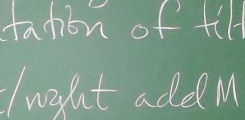BIREP – Representations of finite dimensional algebras at Bielefeld# Bielefeld Projects of the DFG Priority Programme "Representation Theory"

The Priority Programme "Representation Theory" (SPP 1388) was funded for six years (2009 – 2015) by the German Research Foundation (DFG). Within that framework four projects were based in Bielefeld:

### Matrix Factorizations and Complete Intersection Rings

Jesse Burke

The Homological Mirror Symmetry conjecture, proposed by Kontsevich in 1994 as an explanation for a duality between Calabi-Yau three-folds observed in string theory, has had a profound impact on mathematics. In the course of his work on this conjecture Kontsevich rediscovered a construction in commutative algebra known as a matrix factorization. This construction was originally discovered by Eisenbud in 1980 and he used it to describe free resolutions over hypersurface rings. They have since been a standard tool in the representation theory of maximal Cohen-Macaulay modules. This connection between mathematics and physics afforded by matrix factorizations is just beginning to be explored. In this project we would work on several different problems over hypersurface rings, and more generally complete intersection rings, that are related to matrix factorizations and the above connection.

### Spectral Theory of Green Functors and other Commutative 2-Rings

Ivo Dell'Ambrogio

Let G be a finite group. Every Green functor for G – such as the Burnside, the cohomology, or the representation Green functor – captures some aspect of the representation theory of G. If the Green functor is commutative, as in the previous examples, then it admits a symmetric tensor product of its modules, and its derived category is a tensor triangulated category.

Our first goal is to understand the extent to which this is like the derived category of a commutative ring. In particular we aim at proving a classification theorem for its thick subcategories of perfect complexes, in terms of subsets of a suitable topological space, the spectrum of the Green functor; this result should generalize the classification for commutative rings (the case G=1) and Quillen stratification in modular representation theory.

Our second goal lies in developing the proof methods themselves, i.e. new techniques of tensor triangular geometry adapted to Mackey and Green functors. We also wish to explore the natural context for this enterprise, namely presheaves over small symmetric tensor categories, or "commutative 2-rings".

Our third goal is to provide applications to G-equivariant stable homotopy (via the Burnside ring Green functor) and to G-equivariant KK-theory (via the representation ring Green functor).

### Quiver Representations, Singularity Categories and Monoidal Structures

Henning Krause

Representations of algebras are studied from various directions, involving monoidal and triangulated structures. One goal is to describe in terms of generators and relations representation rings and monoids of projections functors for representations of quivers. Another goal is to classify thick and localising subcategories of triangulated singularity categories. This involves continuous and discrete parametrisations, reflecting the existing monoidal or combinatorial structures. The choice of singularity categories is motivated by connections with the representation theory of finite groups, the study of matrix factorisations, and applications in algebraic geometry, including weighted projective lines.

### Toric Structures in Derived Categories and Representations of Algebras

Markus Perling

In this project we want to construct non-commutative coordinatizations of algebraic varieties by construction of exceptional sequences. On a given variety X, an exceptional sequence is a sequence of coherent sheaves satisfying certain conditions of cohomology vanishing. The endomorphism ring of the direct sum of such a sequence forms a non-commutative coordinate system for X in the sense that the derived category of X, i.e. the category of complexes of coherent sheaves on X, is equivalent to the derived category of modules over the endomorphism algebra. This connection serves as a bridge between geometry and the representation theory of algebras and has attracted much interest in recent years, not only in mathematics but also in the context of superstring theory.

Recent results indicate that this correspondence is linked in a deep and unexpected way to the geometry of toric varieties. In this project we want to improve our understanding of this link and we want to use toric geometry to give more general constructions of exceptional sequences and their associated endomorphism rings.